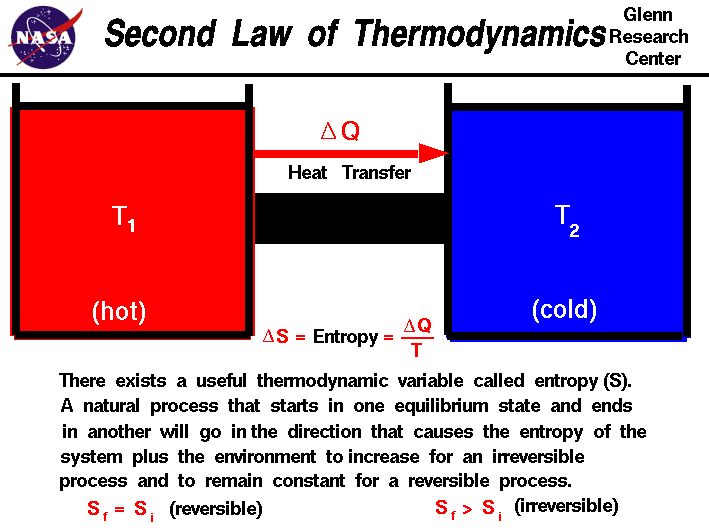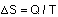القانون الثاني في الديناميكا الحرارية The second law of thermodynamics

### EntropyA measure of the level of disorder of a system is entropy, represented by S. Although it's difficult to measure the total entropy of a system, it's generally fairly easy to measure changes in entropy. For a thermodynamic system involved in a heat transfer of size Q at a temperature T , a change in entropy can be measured by:The second law of thermodynamics can be stated in terms of entropy. If a reversible process occurs, there is no net change in entropy. In an irreversible process, entropy always increases, so the change in entropy is positive. The total entropy of the universe is continually increasing.

There is a strong connection between probability and entropy. This applies to thermodynamic systems like a gas in a box as well as to tossing coins. If you have four pennies, for example, the likelihood that all four will land heads up is relatively small. It is six times more likely that you'll get two heads and two tails. The two heads - two tails state is the most likely, shows the most disorder, and has the highest entropy. Four heads is less likely, has the most order, and the lowest entropy. If you tossed more coins, it would be even less likely that they'd all land heads up, and even more likely that you'd end up with close to the same number of heads as tails.

With a gas in a box, the probability that all the gas molecules are in one corner of the box at the same time is very small (for a typical box full of 1020 molecules or more, incredibly small): this is therefore a low entropy state. It is much more likely that the molecules are randomly distributed around the box, and are moving in random directions; this high disorder state is a considerably higher entropy state. The second law doesn't rule out all the molecules ending up in one corner, but it means it's far more likely that the molecules will be randomly distributed, and to move towards a random distribution from an orderly distribution, asopposed to the other way around.

المصدر

### فيديو

«الرجوع للقسم»
تصميم وبرمجة المتحدة لخدمات الانترنت

جميع الحقوق محفوظة © لموقع لغة الروح